# 【深度学习系列】卷积神经网络CNN原理详解(一)——基本原理

为什么要用神经网络？

• 特征提取的高效性。

• 数据格式的简易性

• 参数数目的少量性

有哪些应用？

详细数学推导

优缺点

传统神经网络的劣势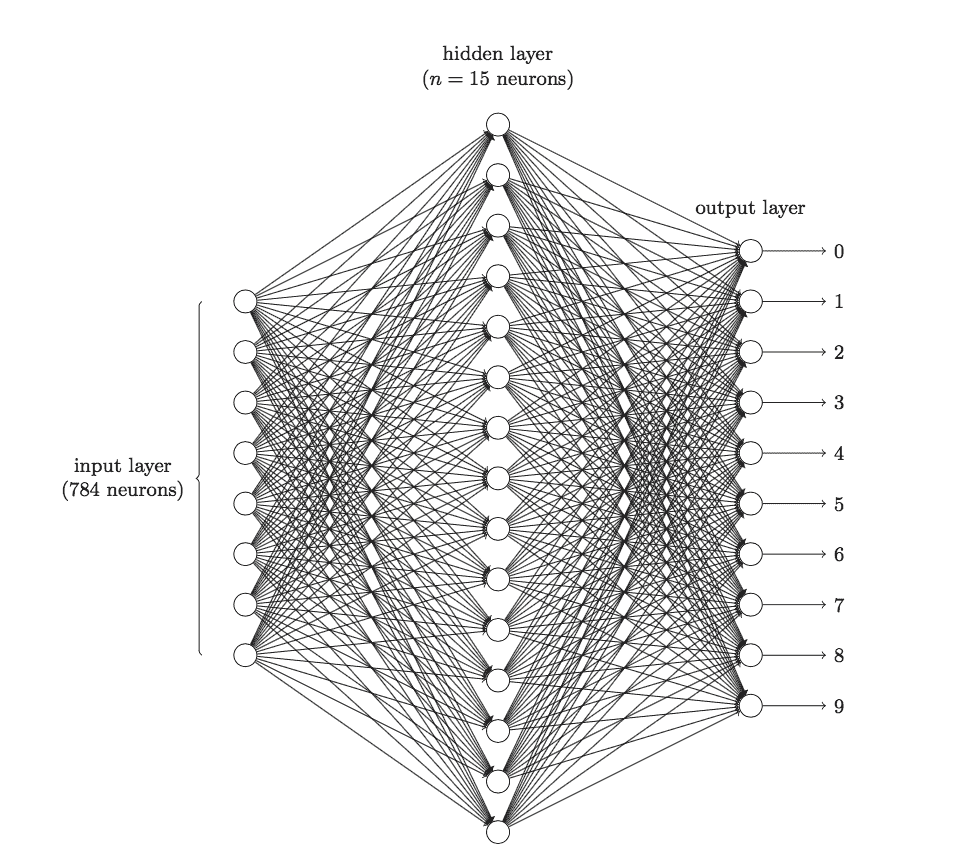卷积神经网络是什么？

三个基本层

• 卷积层（Convolutional Layer）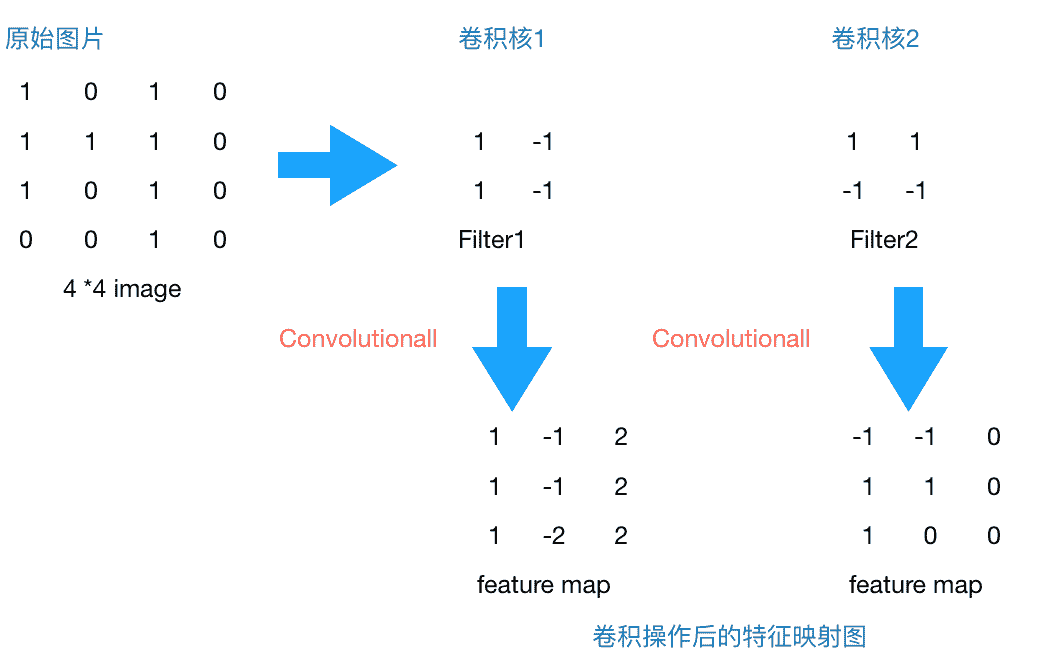feature_map1(1,1) = 1*1 + 0*(-1) + 1*1 + 1*(-1) = 1
feature_map1(1,2) = 0*1 + 1*(-1) + 1*1 + 1*(-1) = -1
...
feature_map1(3,3) = 1*1 + 0*(-1) + 1*1 + 0*(-1) = 2


conv_pool_1 = paddle.networks.simple_img_conv_pool(
input=img,
filter_size=3,
num_filters=2,
num_channel=1,
pool_stride=1,


def simple_img_conv_pool(input,
filter_size,
num_filters,
pool_size,
name=None,
pool_type=None,
act=None,
groups=1,
conv_stride=1,
bias_attr=None,
num_channel=None,
param_attr=None,
shared_bias=True,
conv_layer_attr=None,
pool_stride=1,
pool_layer_attr=None):
"""
Simple image convolution and pooling group.
Img input => Conv => Pooling => Output.
:param name: group name.
:type name: basestring
:param input: input layer.
:type input: LayerOutput
:param filter_size: see img_conv_layer for details.
:type filter_size: int
:param num_filters: see img_conv_layer for details.
:type num_filters: int
:param pool_size: see img_pool_layer for details.
:type pool_size: int
:param pool_type: see img_pool_layer for details.
:type pool_type: BasePoolingType
:param act: see img_conv_layer for details.
:type act: BaseActivation
:param groups: see img_conv_layer for details.
:type groups: int
:param conv_stride: see img_conv_layer for details.
:type conv_stride: int
:param conv_padding: see img_conv_layer for details.
:param bias_attr: see img_conv_layer for details.
:type bias_attr: ParameterAttribute
:param num_channel: see img_conv_layer for details.
:type num_channel: int
:param param_attr: see img_conv_layer for details.
:type param_attr: ParameterAttribute
:param shared_bias: see img_conv_layer for details.
:type shared_bias: bool
:param conv_layer_attr: see img_conv_layer for details.
:type conv_layer_attr: ExtraLayerAttribute
:param pool_stride: see img_pool_layer for details.
:type pool_stride: int
:param pool_padding: see img_pool_layer for details.
:param pool_layer_attr: see img_pool_layer for details.
:type pool_layer_attr: ExtraLayerAttribute
:return: layer's output
:rtype: LayerOutput
"""
_conv_ = img_conv_layer(
name="%s_conv" % name,
input=input,
filter_size=filter_size,
num_filters=num_filters,
num_channels=num_channel,
act=act,
groups=groups,
stride=conv_stride,
bias_attr=bias_attr,
param_attr=param_attr,
shared_biases=shared_bias,
layer_attr=conv_layer_attr)
return img_pool_layer(
name="%s_pool" % name,
input=_conv_,
pool_size=pool_size,
pool_type=pool_type,
stride=pool_stride,
layer_attr=pool_layer_attr)


def simple_img_conv_pool(input,
num_filters,
filter_size,
pool_size,
pool_stride,
act,
pool_type='max',
main_program=None,
startup_program=None):
conv_out = layers.conv2d(
input=input,
num_filters=num_filters,
filter_size=filter_size,
act=act,
main_program=main_program,
startup_program=startup_program)

pool_out = layers.pool2d(
input=conv_out,
pool_size=pool_size,
pool_type=pool_type,
pool_stride=pool_stride,
main_program=main_program,
startup_program=startup_program)
return pool_out


conv2d:

def conv2d(input,
num_filters,
name=None,
filter_size=[1, 1],
act=None,
groups=None,
stride=[1, 1],
bias_attr=None,
param_attr=None,
main_program=None,
startup_program=None):
helper = LayerHelper('conv2d', **locals())
dtype = helper.input_dtype()

num_channels = input.shape
if groups is None:
num_filter_channels = num_channels
else:
if num_channels % groups is not 0:
raise ValueError("num_channels must be divisible by groups.")
num_filter_channels = num_channels / groups

if isinstance(filter_size, int):
filter_size = [filter_size, filter_size]
if isinstance(stride, int):
stride = [stride, stride]

input_shape = input.shape
filter_shape = [num_filters, num_filter_channels] + filter_size

std = (2.0 / (filter_size**2 * num_channels))**0.5
filter = helper.create_parameter(
attr=helper.param_attr,
shape=filter_shape,
dtype=dtype,
initializer=NormalInitializer(0.0, std, 0))
pre_bias = helper.create_tmp_variable(dtype)

helper.append_op(
type='conv2d',
inputs={
'Input': input,
'Filter': filter,
},
outputs={"Output": pre_bias},
attrs={'strides': stride,
'groups': groups})

pre_act = helper.append_bias_op(pre_bias, 1)

return helper.append_activation(pre_act)


pool2d:

def pool2d(input,
pool_size,
pool_type,
pool_stride=[1, 1],
global_pooling=False,
main_program=None,
startup_program=None):
if pool_type not in ["max", "avg"]:
raise ValueError(
"Unknown pool_type: '%s'. It can only be 'max' or 'avg'.",
str(pool_type))
if isinstance(pool_size, int):
pool_size = [pool_size, pool_size]
if isinstance(pool_stride, int):
pool_stride = [pool_stride, pool_stride]

helper = LayerHelper('pool2d', **locals())
dtype = helper.input_dtype()
pool_out = helper.create_tmp_variable(dtype)

helper.append_op(
type="pool2d",
inputs={"X": input},
outputs={"Out": pool_out},
attrs={
"poolingType": pool_type,
"ksize": pool_size,
"globalPooling": global_pooling,
"strides": pool_stride,
})

return pool_out


import copy
import itertools

from paddle.v2.framework.framework import Variable, g_main_program, \
g_startup_program, unique_name, Program
UniformInitializer

class LayerHelper(object):
def __init__(self, layer_type, **kwargs):
self.kwargs = kwargs
self.layer_type = layer_type
name = self.kwargs.get('name', None)
if name is None:
self.kwargs['name'] = unique_name(self.layer_type)

@property
def name(self):
return self.kwargs['name']

@property
def main_program(self):
prog = self.kwargs.get('main_program', None)
if prog is None:
return g_main_program
else:
return prog

@property
def startup_program(self):
prog = self.kwargs.get('startup_program', None)
if prog is None:
return g_startup_program
else:
return prog

def append_op(self, *args, **kwargs):
return self.main_program.current_block().append_op(*args, **kwargs)

def multiple_input(self, input_param_name='input'):
inputs = self.kwargs.get(input_param_name, [])
type_error = TypeError(
"Input of {0} layer should be Variable or sequence of Variable".
format(self.layer_type))
if isinstance(inputs, Variable):
inputs = [inputs]
elif not isinstance(inputs, list) and not isinstance(inputs, tuple):
raise type_error
else:
for each in inputs:
if not isinstance(each, Variable):
raise type_error
return inputs

def input(self, input_param_name='input'):
inputs = self.multiple_input(input_param_name)
if len(inputs) != 1:
raise "{0} layer only takes one input".format(self.layer_type)
return inputs

@property
def param_attr(self):
default = {'name': None, 'initializer': UniformInitializer()}
actual = self.kwargs.get('param_attr', None)
if actual is None:
actual = default
for default_field in default.keys():
if default_field not in actual:
actual[default_field] = default[default_field]
return actual

def bias_attr(self):
default = {'name': None, 'initializer': ConstantInitializer()}
bias_attr = self.kwargs.get('bias_attr', None)
if bias_attr is True:
bias_attr = default

if isinstance(bias_attr, dict):
for default_field in default.keys():
if default_field not in bias_attr:
bias_attr[default_field] = default[default_field]
return bias_attr

def multiple_param_attr(self, length):
param_attr = self.param_attr
if isinstance(param_attr, dict):
param_attr = [param_attr]

if len(param_attr) != 1 and len(param_attr) != length:
raise ValueError("parameter number mismatch")
elif len(param_attr) == 1 and length != 1:
tmp = [None] * length
for i in xrange(length):
tmp[i] = copy.deepcopy(param_attr)
param_attr = tmp
return param_attr

def iter_inputs_and_params(self, input_param_name='input'):
inputs = self.multiple_input(input_param_name)
param_attrs = self.multiple_param_attr(len(inputs))
for ipt, param_attr in itertools.izip(inputs, param_attrs):
yield ipt, param_attr

def input_dtype(self, input_param_name='input'):
inputs = self.multiple_input(input_param_name)
dtype = None
for each in inputs:
if dtype is None:
dtype = each.data_type
elif dtype != each.data_type:
raise ValueError("Data Type mismatch")
return dtype

def create_parameter(self, attr, shape, dtype, suffix='w',
initializer=None):
# Deepcopy the attr so that parameters can be shared in program
attr_copy = copy.deepcopy(attr)
if initializer is not None:
attr_copy['initializer'] = initializer
if attr_copy['name'] is None:
attr_copy['name'] = unique_name(".".join([self.name, suffix]))
self.startup_program.global_block().create_parameter(
dtype=dtype, shape=shape, **attr_copy)
return self.main_program.global_block().create_parameter(
name=attr_copy['name'], dtype=dtype, shape=shape)

def create_tmp_variable(self, dtype):
return self.main_program.current_block().create_var(
name=unique_name(".".join([self.name, 'tmp'])),
dtype=dtype,
persistable=False)

def create_variable(self, *args, **kwargs):
return self.main_program.current_block().create_var(*args, **kwargs)

def create_global_variable(self, persistable=False, *args, **kwargs):
return self.main_program.global_block().create_var(
*args, persistable=persistable, **kwargs)

def set_variable_initializer(self, var, initializer):
assert isinstance(var, Variable)
self.startup_program.global_block().create_var(
name=var.name,
type=var.type,
dtype=var.data_type,
shape=var.shape,
persistable=True,
initializer=initializer)

def append_bias_op(self, input_var, num_flatten_dims=None):
"""
Append bias operator and return its output. If the user does not set
bias_attr, append_bias_op will return input_var

:param input_var: the input variable. The len(input_var.shape) is larger
or equal than 2.
:param num_flatten_dims: The input tensor will be flatten as a matrix
matrix.shape = product(input_var.shape[0:num_flatten_dims]), product(
input_var.shape[num_flatten_dims:])
"""
if num_flatten_dims is None:
num_flatten_dims = self.kwargs.get('num_flatten_dims', None)
if num_flatten_dims is None:
num_flatten_dims = 1

size = list(input_var.shape[num_flatten_dims:])
bias_attr = self.bias_attr()
if not bias_attr:
return input_var

b = self.create_parameter(
attr=bias_attr, shape=size, dtype=input_var.data_type, suffix='b')
tmp = self.create_tmp_variable(dtype=input_var.data_type)
self.append_op(
inputs={'X': [input_var],
'Y': [b]},
outputs={'Out': [tmp]})
return tmp

def append_activation(self, input_var):
act = self.kwargs.get('act', None)
if act is None:
return input_var
if isinstance(act, basestring):
act = {'type': act}
tmp = self.create_tmp_variable(dtype=input_var.data_type)
act_type = act.pop('type')
self.append_op(
type=act_type,
inputs={"X": [input_var]},
outputs={"Y": [tmp]},
attrs=act)
return tmp


: 1.此处的卷积运算是两个卷积核大小的矩阵的内积运算，不是矩阵乘法。即相同位置的数字相乘再相加求和。不要弄混淆了。

2.卷积核的公式有很多，这只是最简单的一种。我们所说的卷积核在数字信号处理里也叫滤波器，那滤波器的种类就多了，均值滤波器，高斯滤波器，拉普拉斯滤波器等等，不过，不管是什么滤波器，都只是一种数学运算，无非就是计算更复杂一点。

3.每一层的卷积核大小和个数可以自己定义，不过一般情况下，根据实验得到的经验来看，会在越靠近输入层的卷积层设定少量的卷积核，越往后，卷积层设定的卷积核数目就越多。具体原因大家可以先思考一下，小结里会解释原因。

• 池化层（Pooling Layer）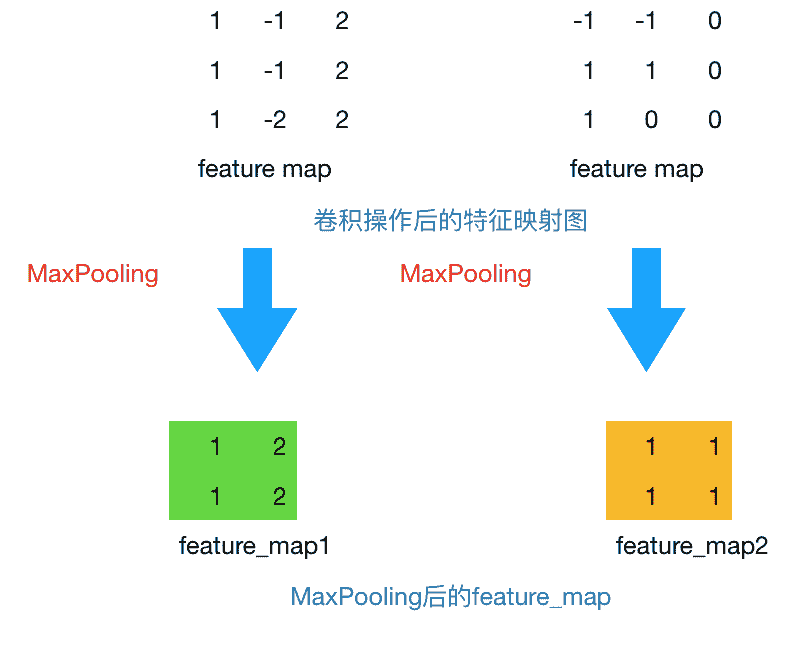• MaxPooling: 取滑动窗口里最大的值
• AveragePooling: 取滑动窗口内所有值的平均值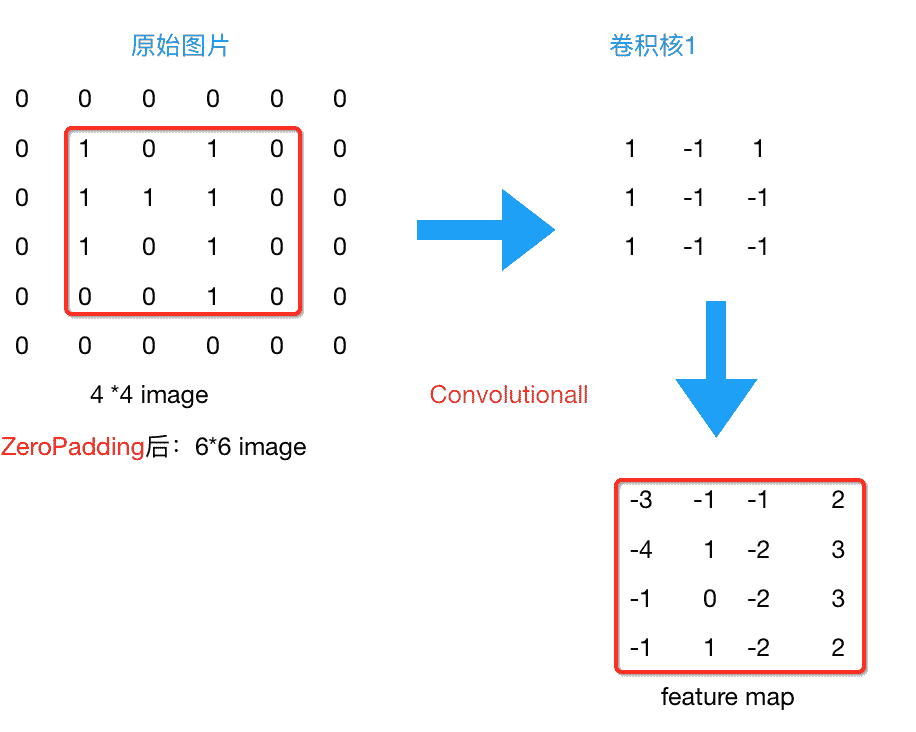• Flatten层 & Fully Connected Layer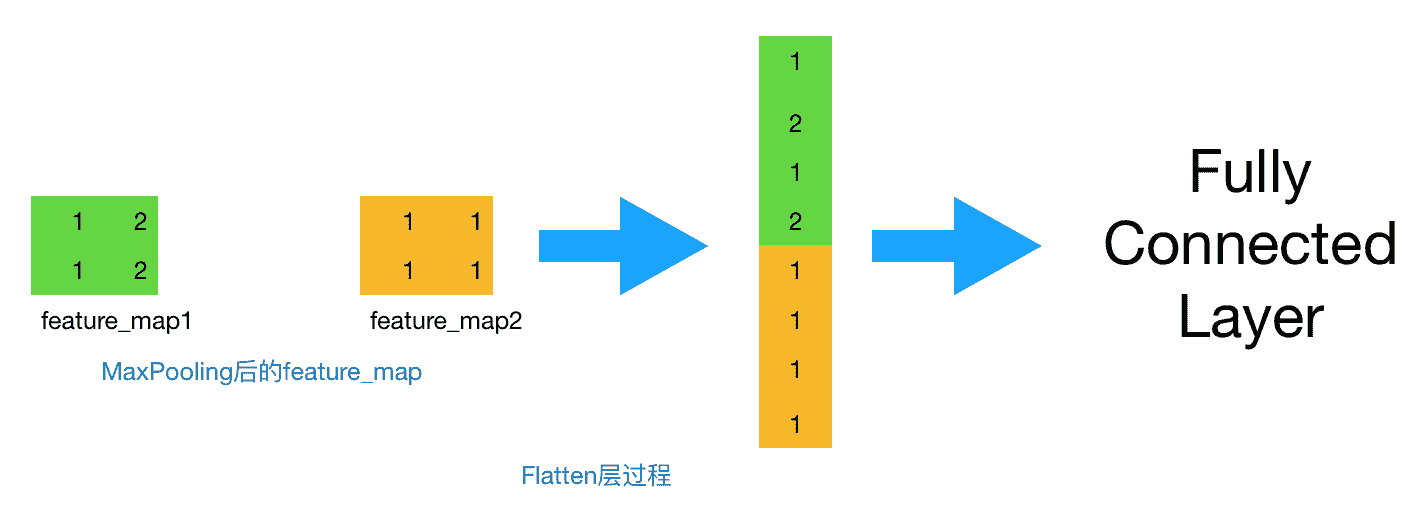• 小结

1.卷积核的尺寸必须为正方形吗？可以为长方形吗？如果是长方形应该怎么计算？

2.卷积核的个数如何确定？每一层的卷积核的个数都是相同的吗？

3.步长的向右和向下移动的幅度必须是一样的吗？

1.卷积核的尺寸不一定非得为正方形。长方形也可以，只不过通常情况下为正方形。如果要设置为长方形，那么首先得保证这层的输出形状是整数，不能是小数。如果你的图像是边长为 28 的正方形。那么卷积层的输出就满足 [ (28 - kernel_size)/ stride ] + 1 ，这个数值得是整数才行，否则没有物理意义。譬如，你算得一个边长为 3.6 的 feature map 是没有物理意义的。 pooling 层同理。FC 层的输出形状总是满足整数，其唯一的要求就是整个训练过程中 FC 层的输入得是定长的。如果你的图像不是正方形。那么在制作数据时，可以缩放到统一大小（非正方形），再使用非正方形的 kernel_size 来使得卷积层的输出依然是整数。总之，撇开网络结果设定的好坏不谈，其本质上就是在做算术应用题: 如何使得各层的输出是整数。

2.由经验确定。通常情况下，靠近输入的卷积层，譬如第一层卷积层，会找出一些共性的特征，如手写数字识别中第一层我们设定卷积核个数为5个，一般是找出诸如"横线"、“竖线”、“斜线”等共性特征，我们称之为basic feature，经过max pooling后，在第二层卷积层，设定卷积核个数为20个，可以找出一些相对复杂的特征，如“横折”、“左半圆”、“右半圆”等特征，越往后，卷积核设定的数目越多，越能体现label的特征就越细致，就越容易分类出来，打个比方，如果你想分类出“0”的数字，你看到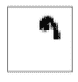这个特征，能推测是什么数字呢？只有越往后，检测识别的特征越多，试过能识别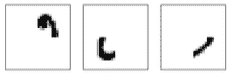这几个特征，那么我就能够确定这个数字是“0”。

3.有stride_w和stride_h，后者表示的就是上下步长。如果用stride，则表示stride_h=stride_w=stride。

*　　*手写数字识别的CNN网络结构

def convolutional_neural_network_org(img):
# first conv layer
input=img,
filter_size=3,
num_filters=20,
num_channel=1,
pool_size=2,
pool_stride=2,
# second conv layer
input=conv_pool_1,
filter_size=5,
num_filters=50,
num_channel=20,
pool_size=2,
pool_stride=2,
# fully-connected layer
return predict


conv1----> conv2---->fully Connected layer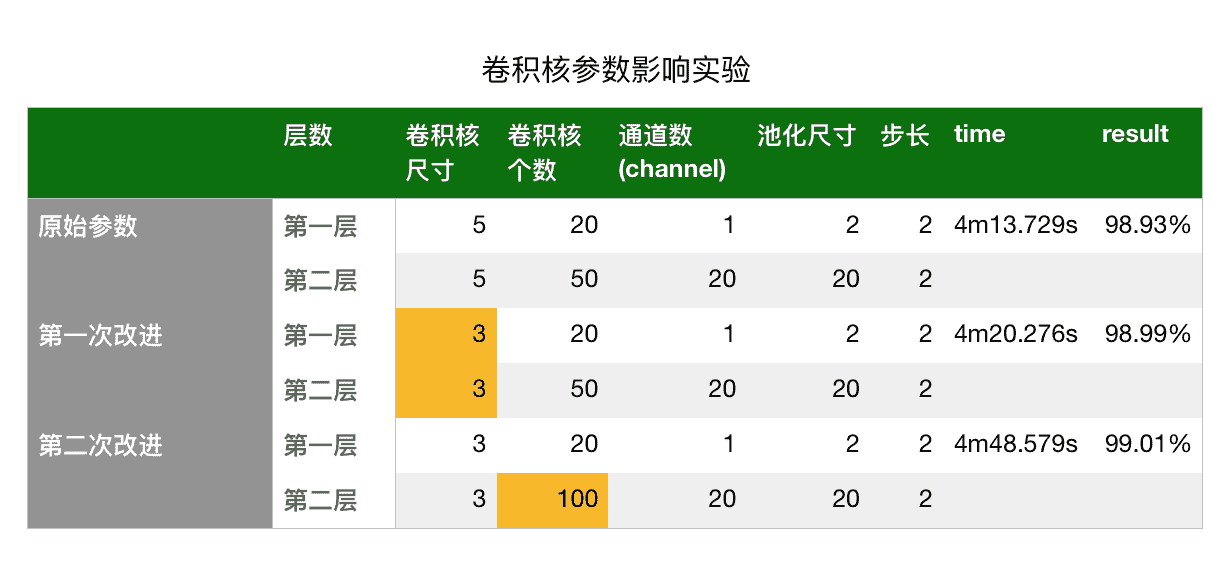• 第一次改进: 仅改变第一层与第二层的卷积核数目的大小，其他保持不变。可以看到结果提升了0.06%
• 第二次改进: 保持3*3的卷积核大小，仅改变第二层的卷积核数目，其他保持不变，可以看到结果相较于原始参数提升了0.08%

• 为什么卷积神经网络更适合于图像分类？相比于传统的神经网络优势在哪里？
• 卷积层中的卷积过程是如何计算的？为什么卷积核是有效的？
• 卷积核的个数如何确定？应该选择多大的卷积核对于模型来说才是有效的？尺寸必须为正方形吗？如果是长方形因该怎么做？
• 步长的大小会对模型的效果产生什么样的影响？垂直方向和水平方向的步长是否得设定为相同的？
• 为什么要采用池化层，Max Pooling有什么好处？

• 为什么改变卷积核的大小能够提高结果的准确率？卷积核大小对于分类结果是如何影响的？
• 卷积核的参数是怎么求的？一开始随机定义一个，那么后来是如何训练才能使这个卷积核识别某些特定的特征呢？
• 11的卷积核有意义吗？为什么有些网络层结构里会采用11的卷积核？

• 卷积核的参数如何确定？随机初始化一个数值后，是如何训练得到一个能够识别某些特征的卷积核的？
• CNN是如何进行反向传播的？
• 如何调整CNN里的参数？
• 如何设计最适合的CNN网络结构？